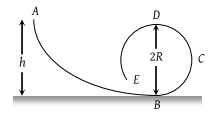Which one of the following is not a conservative force

(1) Gravitational force

(2) Electrostatic force between two charges

(3) Magnetic force between two magnetic dipoles

(4) Frictional force

Concept Questions :-

Work-Energy theorem
High Yielding Test Series + Question Bank - NEET 2020

Difficulty Level:

Work done in raising a box depends on

(1) How fast it is raised

(2) The strength of the man

(3) The height by which it is raised

(4) None of the above

Concept Questions :-

Gravitational Potential energy
High Yielding Test Series + Question Bank - NEET 2020

Difficulty Level:

A frictionless track ABCDE ends in a circular loop of radius R. A body slides down the track from point A which is at a height h = 5 cm. Maximum value of R for the body to successfully complete the loop is(1) 5 cm

(2) $\frac{15}{4}\text{\hspace{0.17em}}cm$

(3) $\frac{10}{3}\text{\hspace{0.17em}}cm$

(4) 2 cm

Concept Questions :-

Work-Energy theorem
High Yielding Test Series + Question Bank - NEET 2020

Difficulty Level:

If the water falls from a dam into a turbine wheel 19.6 m below, then the velocity of water at the turbine is (g = 9.8 m/s2)

(1) 9.8 m/s

(2) 19.6 m/s

(3) 39.2 m/s

(4) 98.0 m/s

Concept Questions :-

Gravitational Potential energy
High Yielding Test Series + Question Bank - NEET 2020

Difficulty Level:

Two identical cylindrical vessels with their bases at the same level each contain a liquid of density; $\rho$. The height of the liquid in one vessel is h1 and that in the other vessel is h2 $\left({h}_{1}>{h}_{2}\right)$.The area of either base is A. The work done by gravity in equalizing the levels when the two vessels are connected is :

(1) $\left({h}_{1}-{h}_{2}\right)g\rho$

(2) $\left({h}_{1}-{h}_{2}\right)gA\rho$

(3) $\frac{1}{2}{\left({h}_{1}-{h}_{2}\right)}^{2}gA\rho$

(4) $\frac{1}{4}{\left({h}_{1}-{h}_{2}\right)}^{2}gA\rho$

Concept Questions :-

Gravitational Potential energy
High Yielding Test Series + Question Bank - NEET 2020

Difficulty Level:

If the increase in the kinetic energy of a body is 22%, then the increase in the momentum will be

(1) 22%

(2) 44%

(3) 10%

(4) 300%

High Yielding Test Series + Question Bank - NEET 2020

Difficulty Level:

If a body of mass 200 g falls from a height 200 m and its total P.E. is converted into K.E. at the point of contact of the body with earth surface, then what is the decrease in P.E. of the body at the contact (g = 10 m/s2)

(1) 200 J

(2) 400 J

(3) 600 J

(4) 900 J

High Yielding Test Series + Question Bank - NEET 2020

Difficulty Level:

If momentum is increased by 20%, then K.E. increases by

(1) 44%

(2) 55%

(3) 66%

(4) 77%

High Yielding Test Series + Question Bank - NEET 2020

Difficulty Level:

The kinetic energy of a body of mass 2 kg and momentum of 2 Ns is

(1) 1 J

(2) 2 J

(3) 3 J

(4) 4 J

High Yielding Test Series + Question Bank - NEET 2020

Difficulty Level:

The decrease in the potential energy of a ball of mass 20 kg which falls from a height of 50 cm is

(1) 968 J

(2) 98 J

(3) 1980 J

(4) None of these

Concept Questions :-

Gravitational Potential energy